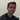# NaN (Not a Number)Written by John D. Johnson II who lives and works in Utah.

# Long-Division Proof

December 11, 2020

## Background:

I read a comment on Hacker News sometime December 2019 about the general long division algorithm being unintuitive. This guy stated his credentials and the comment after his said surely he could come up with a proof in around 15-30 minutes, so I like Math and I like to think I'm at least somewhat good at it for not being a mathematician, so I challenged myself to write up a proof just for fun. Although I wrote out the first sketch of the proof that night it took me until now to do a writeup of it.

## Justification for a proof

You might ask well why a proof, and I just have to say I feel a proof is the best way to see something intuitively. Here is my attempt at proving the long division algorithm. If you notice any mistakes please let me know on twitter or on a github issue on my blog or by any other means you can contact me with.

First I'll show an example of the long-division algorithm the way I learned it in school. This is honestly what I first wrote down to get the correct mental model of the algorithm.

### Example

So, first we will divide 128 by 5 just as an example.

We set up the equation:

$\begin{array}{l} \phantom{5;1}{} \\ 5\overline{\smash{)}128} \\ \end{array}$

We first notice five doesn't go into 1 so we do our first division of five on 12 instead five goes into 12 two times so we write two on top and subtract $2\times5$ or $10$:

$\begin{array}{l} \phantom{5;1}{2} \\ 5\overline{\smash{)}128} \\ \phantom{}{-\underline{10}} \end{array}$

that subtraction produces two:

$\begin{array}{l} \phantom{5;1}{2} \\ 5\overline{\smash{)}128} \\ \phantom{}{-\underline{10}} \\ \phantom{-1}{2} \end{array}$

Then we bring down the 8:

$\begin{array}{l} \phantom{5;1}{2} \\ 5\overline{\smash{)}128} \\ \phantom{}{-\underline{10}\smash{\downarrow}} \\ \phantom{-1}{28} \end{array}$

and repeat until we get:

$\begin{array}{l} \phantom{5;1}{25\frac{3}{5}} \\ 5\overline{\smash{)}128\phantom{3}} \\ \phantom{}{-\underline{10}\smash{\downarrow}} \\ \phantom{-1}{28} \\ \phantom{1}{-\underline{25}} \\ \phantom{-12}{3} \end{array}$

## Clearing up Some Hand-wavyness

So, there are some parts there that may seem a little mysterious and hand-wavy, so lets start by clearing those up. First of all at this step where we subtract 10:

$\begin{array}{l} \phantom{5;1}{2} \\ 5\overline{\smash{)}128} \\ \phantom{}{-\underline{10}} \end{array}$

We are really or atleast can really divide 5 from 12, if you want to think of it that way 5 from 12*10 which is 120. and subtracting 100 which five goes into 100 twenty times.

$\begin{array}{l} \phantom{5;1}{20} \\ 5\overline{\smash{)}128} \\ \phantom{}{-\underline{100}} \end{array}$

This mental model will come in handy for our proof. There are some other mental models that will help us for example notice that the right side of this equation is our result rewritten:

$25\frac{3}{5}=20 + 5 + \frac{3}{5}$

Or we could rewrite our division as

$\frac{128}{5} = \frac{1}{5}\cdot (100 + 20 + 8)$

## First Proof Attempt

WARNING: this is gonna get a bit more math-intensive than the rest of the post after this point...

This was my first attempt to the proof which I did that night upon reading the HN comment, though it appears correct to me, I'm not sure I like it as much as the second Proof Attempt I have below.

Thus we see the general form is like the following where all numbers, $n,x_1,x_2,\ldots,x_y$, are of the set $\{0,1,2,3,\ldots\}$ and $m$ is of the set $\{1,2,3,\ldots\}$.

$\begin{array}{l} \phantom{5;}x_1+x_2+\ldots+x_y+\frac{n-mx_1-mx_2-\ldots-mx_y}{m} \\ m\overline{\smash{)}\phantom{1}n} \\ -\underline{mx_1} \\ \phantom{-}n-mx_1 \\ -\underline{mx_2} \\ \phantom{-}n-mx_1-mx_2 \\ -\underline{\ldots} \\ \phantom{-}n-mx_1-mx_2-\ldots-mx_y \\ \end{array}$

So, we know $n-mx_1-mx_2-\ldots-mx_y$ is the remainder, and $x_1+x_2+\ldots+x_y+\frac{n-mx_1-mx_2-\ldots-mx_y}{m}$ is the result.

Thus we need to prove equality of:

$\frac{n}{m}=x_1+x_2+\ldots+x_y+\frac{n-mx_1-mx_2-\ldots-mx_y}{m}$

Let's multiply each side of the equation by $m$ and then prove equality by simplifying the right hand side.

$m\cdot\frac{n}{m}=m\cdot(x_1+x_2+\ldots+x_y+\frac{n-mx_1-mx_2-\ldots-mx_y}{m})$

$n=m\cdot(x_1+x_2+\ldots+x_y)+m\cdot(\frac{n-mx_1-mx_2-\ldots-mx_y}{m})$

$n=mx_1+mx_2+\ldots+mx_y+n-mx_1-mx_2-\ldots-mx_y$

$n=n$

## Proof Attempt 2

This was my second and in my opinion more elegant approach that I wrote up sometime well I was reviewing my other proof to be posted. It's very similar, but better in my opinion as I did not have to manipulate both sides of the equation (because generally manipulating both sides can get you into false proof territory.)

We could do this one other way where we start at:

$\frac{n}{m}=x_1+x_2+\ldots+x_y+\frac{n-mx_1-mx_2-\ldots-mx_y}{m}$

We could just simplify the right hand side of the equation to make it equal the left hand side.

(Distributive property of division and subtraction)

$\frac{n}{m}=x_1+x_2+\ldots+x_y+\frac{n}{m}-\frac{mx_1}{m}-\frac{mx_2}{m}-\ldots-\frac{mx_y}{m}$

(Cancelling out like terms with division)

$\frac{n}{m}=x_1+x_2+\ldots+x_y+\frac{n}{m}-x_1-x_2-\ldots-x_y$

(Commutative property of addition/subtraction)

$\frac{n}{m}=(x_1-x_1)+(x_2-x_2)+\ldots+(x_y-x_y)+\frac{n}{m}$

(Cancelling out like terms with subtraction)

$\frac{n}{m}=\frac{n}{m}$

$\blacksquare$Written by John D. Johnson II who lives and works in Utah.